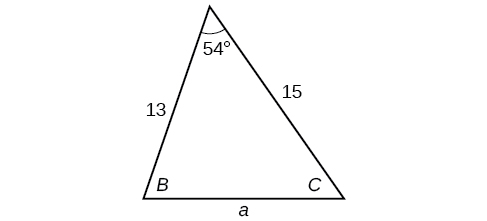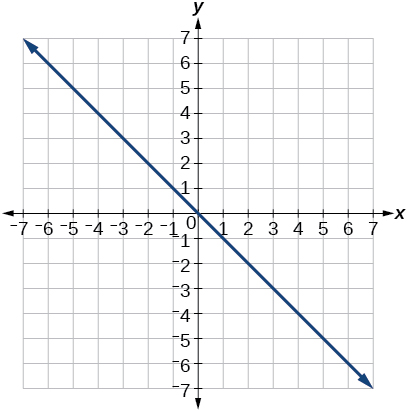$$\newcommand{\id}{\mathrm{id}}$$ $$\newcommand{\Span}{\mathrm{span}}$$ $$\newcommand{\kernel}{\mathrm{null}\,}$$ $$\newcommand{\range}{\mathrm{range}\,}$$ $$\newcommand{\RealPart}{\mathrm{Re}}$$ $$\newcommand{\ImaginaryPart}{\mathrm{Im}}$$ $$\newcommand{\Argument}{\mathrm{Arg}}$$ $$\newcommand{\norm}{\| #1 \|}$$ $$\newcommand{\inner}{\langle #1, #2 \rangle}$$ $$\newcommand{\Span}{\mathrm{span}}$$

# 8.R: Further Applications of Trigonometry (Review)

$$\newcommand{\vecs}{\overset { \rightharpoonup} {\mathbf{#1}} }$$ $$\newcommand{\vecd}{\overset{-\!-\!\rightharpoonup}{\vphantom{a}\smash {#1}}}$$$$\newcommand{\id}{\mathrm{id}}$$ $$\newcommand{\Span}{\mathrm{span}}$$ $$\newcommand{\kernel}{\mathrm{null}\,}$$ $$\newcommand{\range}{\mathrm{range}\,}$$ $$\newcommand{\RealPart}{\mathrm{Re}}$$ $$\newcommand{\ImaginaryPart}{\mathrm{Im}}$$ $$\newcommand{\Argument}{\mathrm{Arg}}$$ $$\newcommand{\norm}{\| #1 \|}$$ $$\newcommand{\inner}{\langle #1, #2 \rangle}$$ $$\newcommand{\Span}{\mathrm{span}}$$ $$\newcommand{\id}{\mathrm{id}}$$ $$\newcommand{\Span}{\mathrm{span}}$$ $$\newcommand{\kernel}{\mathrm{null}\,}$$ $$\newcommand{\range}{\mathrm{range}\,}$$ $$\newcommand{\RealPart}{\mathrm{Re}}$$ $$\newcommand{\ImaginaryPart}{\mathrm{Im}}$$ $$\newcommand{\Argument}{\mathrm{Arg}}$$ $$\newcommand{\norm}{\| #1 \|}$$ $$\newcommand{\inner}{\langle #1, #2 \rangle}$$ $$\newcommand{\Span}{\mathrm{span}}$$

## 8.1: Non-right Triangles: Law of Sines

For the exercises 1-5 assume $$\alpha$$ is opposite side $$a$$, $$\beta$$ is opposite side $$b$$, and $$\gamma$$ is opposite side $$c$$. Solve each triangle, if possible. Round each answer to the nearest tenth.

1) $$\beta =50^{\circ}, a=105, b=45$$

Not possible

2) $$\alpha =43.1^{\circ}, a=184.2, b=242.8$$

3) Solve the triangle.$$C=120^{\circ}, a=23.1, c=34.1$$

4) Find the area of the triangle.

5) A pilot is flying over a straight Highway. He determines the angles of depression to two mile posts $$2.1$$ km apart to be $$25^{\circ}$$ and $$49^{\circ}$$, as shown in the figure below. Find the distance of the plane from point $$A$$ and the elevation of the plane.distance of the plane from point $$A:2.2$$ km, elevation of the plane: $$1.6$$ km

## 8.2: Non-right Triangles - Law of Cosines

1) Solve the triangle, rounding to the nearest tenth, assuming $$\alpha$$ is opposite side $$a$$, $$\beta$$ is opposite side $$b$$, and $$\gamma$$ s opposite side $$c: a=4, b=6,c=8$$$.$

2) Solve the triangle in the Figure below, rounding to the nearest tenth.$$B=71.0^{\circ},C=55.0^{\circ},a=12.8$$

3) Find the area of a triangle with sides of length $$8.3$$, $$6.6$$, and $$9.1$$.

4) To find the distance between two cities, a satellite calculates the distances and angle shown in the Figure below (not to scale). Find the distance between the cities. Round answers to the nearest tenth.$$40.6$$ km

## 8.3: Polar Coordinates

1) Plot the point with polar coordinates $$\left ( 3,\dfrac{\pi }{6} \right )$$.

2) Plot the point with polar coordinates $$\left ( 5,\dfrac{-2\pi }{3} \right )$$.3) Convert $$\left ( 6,\dfrac{-3\pi }{4} \right )$$ to rectangular coordinates.

4) Convert $$\left ( -2,\dfrac{3\pi }{2} \right )$$ to rectangular coordinates.

$$(0,2)$$

5) Convert $$(7,-2)$$ to polar coordinates.

6) Convert $$(-9,-4)$$ to polar coordinates.

$$(9.8489,203.96^{\circ})$$

For the exercises 7-9, convert the given Cartesian equation to a polar equation.

7) $$x=-2$$

8) $$x^2+y^2=64$$

$$r=8$$

9) $$x^2+y^2=-2y$$

For the exercises 10-11, convert the given polar equation to a Cartesian equation.

10) $$r=7\cos \theta$$

$$x^2+y^2=7x$$

11) $$r=\dfrac{-2}{4\cos \theta +\sin \theta }$$

For the exercises 12-13, convert to rectangular form and graph.

12) $$\theta =\dfrac{3\pi }{4}$$

$$y=-x$$13) $$r=5\sec \theta$$

## 8.4: Polar Coordinates - Graphs

For the exercises 1-5, test each equation for symmetry.

1) $$r=4+4\sin \theta$$

symmetric with respect to the line $$\theta =\dfrac{\pi }{2}$$

2) $$r=7$$

3) Sketch a graph of the polar equation $$r=1-5\sin \theta$$. Label the axis intercepts.4) Sketch a graph of the polar equation $$r=5\sin (7\theta )$$.

5) Sketch a graph of the polar equation $$r=3-3\cos \theta$$## 8.5: Polar Form of Complex Numbers

For the exercises 1-2, find the absolute value of each complex number.

1) $$-2+6i$$

2) $$4-3i$$

$$5$$

Write the complex number in polar form.

3) $$5+9i$$

4) $$\dfrac{1}{2}-\dfrac{\sqrt{3}}{2}i$$

$$\mathrm{cis}\left (-\dfrac{\pi }{3} \right )$$

For the exercises 5-6, convert the complex number from polar to rectangular form.

5) $$z=5\mathrm{cis}\left (\dfrac{5\pi }{6} \right )$$

6) $$z=3\mathrm{cis}(40^{\circ})$$

$$2.3+1.9i$$

For the exercises 7-8, find the product $$z_1 z_2$$ in polar form.

7) \begin{align*} z_1 &= 2\mathrm{cis}(89^{\circ})\\ z_2 &= 5\mathrm{cis}(23^{\circ}) \end{align*}

8) \begin{align*} z_1 &= 10\mathrm{cis}\left ( \dfrac{\pi }{6} \right )\\ z_2 &= 6\mathrm{cis}\left ( \dfrac{\pi }{3} \right ) \end{align*}

$$60\mathrm{cis}\left ( \dfrac{\pi }{2} \right )$$

For the exercises 9-10, find the quotient $$\dfrac{z_1}{z_2}$$ in polar form.

9) \begin{align*} z_1 &= 12\mathrm{cis}(55^{\circ})\\ z_2 &= 3\mathrm{cis}(18^{\circ}) \end{align*}

10) \begin{align*} z_1 &= 27\mathrm{cis}\left ( \dfrac{5\pi }{3} \right )\\ z_2 &= 9\mathrm{cis}\left ( \dfrac{\pi }{3} \right ) \end{align*}

$$3\mathrm{cis}\left ( \dfrac{4\pi }{3} \right )$$

For the exercises 11-12, find the powers of each complex number in polar form.

11) Find $$z^4$$ when $$z=2\mathrm{cis}(70^{\circ})$$

12) Find $$z^2$$ when $$z=5\mathrm{cis}\left ( \dfrac{3\pi }{4} \right )$$

$$25\mathrm{cis}\left ( \dfrac{3\pi }{2} \right )$$
13) Evaluate the cube root of $$z$$ when